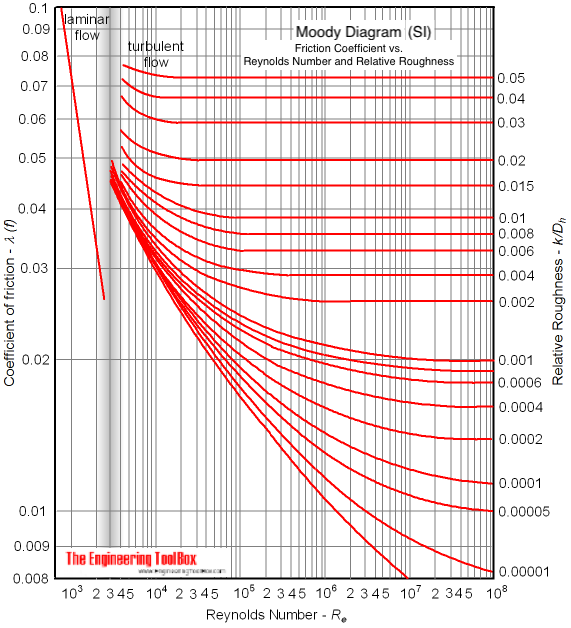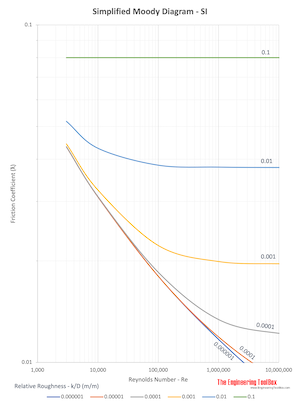Engineering ToolBox - Resources, Tools and Basic Information for Engineering and Design of Technical Applications!

# Colebrook Equation

## Friction loss coefficients in pipes, tubes and ducts.

The friction coefficients used when calculating resistance or pressure loss (or major loss) in ducts, tubes or pipes can be calculated with the Colebrook equation

1 / λ1/2 = -2 log [ 2.51 / (Re λ1/2)  +  (k / dh) / 3.72 ]                           (1)

where

λ = Darcy-Weisbach  friction coefficient

Re = Reynolds Number

k = roughness of duct, pipe or tube surface (m, ft)

dh = hydraulic diameter (m, ft)

The Colebrook equation is only valid at turbulent flow conditions.

Note! - the friction coefficient is involved on both sides of the equation. The equation must therefore be solved by iteration.

The Colebrook equation is generic and can be used to calculate friction coefficients for different kinds of fluid flows - like air ventilation ducts, pipes and tubes with water or oil, compressed air and much more.

### Colebrook Online Calculator

The friction coefficient can be calculated with the online Colebrook calculator below.

The default values used are for turbulent air flow in a 315 mm duct with 6 m/s (0.47 m3/s). The kinematic viscosity used to calculate the Reynolds Number is 15 10-6 m2/s.

Replace the parameters with the appropriate values for your application.

The calculator below can be used to calculate the friction coefficient if velocity and kinematic viscosity of the fluid are known.

The friction coefficient can also be estimated with the Moody diagram (SI based):### Simplified Moody Diagram - SI## Related Topics

• ### Fluid Mechanics

The study of fluids - liquids and gases. Involving velocity, pressure, density and temperature as functions of space and time.
• ### Ventilation

Systems for ventilation and air handling - air change rates, ducts and pressure drops, charts and diagrams and more.

## Related Documents

• ### Darcy-Weisbach Equation - Major Pressure and Head Loss due to Friction

The Darcy-Weisbach equation can be used to calculate the major pressure and head loss due to friction in ducts, pipes or tubes.
• ### Duct Velocity

Calculate velocities in circular and rectangular ducts - imperial and SI-units - online calculator.
• ### Energy Equation - Pressure Loss vs. Head Loss

Calculate pressure loss - or head loss - in ducts, pipes or tubes.
• ### Fluid Flow - Hydraulic Diameter

Calculate hydraulic diameter for pipes and ducts.
• ### Laminar Flow - Friction Coefficients

Calculate friction coefficients for laminar fluid flow.
• ### Moody Diagram

Calculate fluid flow friction coefficients from a Moody diagram.
• ### Natural Draft - Air Flow Volume and Velocity

Air flow - volume and velocity - due to stack or flue effect caused by indoor hot and outdoor cold temperature difference.
• ### Reynolds Number

Introduction and definition of the dimensionless Reynolds Number - online calculators.
• ### Roughness & Surface Coefficients

Surface coefficients that can be used to calculate friction and major pressure loss for fluid flow with surfaces like concrete, galvanized steel, corroded steel and more.

## Engineering ToolBox - SketchUp Extension - Online 3D modeling!

Add standard and customized parametric components - like flange beams, lumbers, piping, stairs and more - to your Sketchup model with the Engineering ToolBox - SketchUp Extension - enabled for use with older versions of the amazing SketchUp Make and the newer "up to date" SketchUp Pro . Add the Engineering ToolBox extension to your SketchUp Make/Pro from the Extension Warehouse !

We don't collect information from our users. More about

## Citation

• The Engineering ToolBox (2008). Colebrook Equation. [online] Available at: https://www.engineeringtoolbox.com/colebrook-equation-d_1031.html [Accessed Day Month Year].

Modify the access date according your visit.

9.29.12